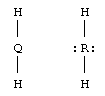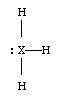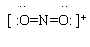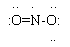## Quiz: Molecular shape (VSEPR); Lewis structures. Answer key.

 1. a.The key points... * QH2 must have no lone pairs. * RH2 must have two lone pairs. (If there were only one lone pair, the molecular shape would still be called "bent". However, the question specified that R should satisfy the octet rule.) * How you arrange the Lewis structures (e.g, whether you show the two H on sides or top/bottom, or together) is irrelevant. A Lewis structure does not show shape; it provides information that allows you then to determine the shape. b. The electron geometry of QH2 is linear. The electron geometry of RH2 is tetrahedral. c. The presence of lone pairs. Lone pairs do affect the underlying geometry. We recognize this when we state the electron geometry, which includes the lone pairs. However, the molecular shape does not include lone pairs, though it is determined by them. d. Nothing. Of course, you would show Cl, with its one bond and three lone pairs, instead of H. But that would not affect any of the substance of parts a-c. The discussion is about the shape around the central atom, Q or R; the nature of the central atom, including its lone pairs, is what matters. e. Be, O. Look at the PT. f. BeH2 and OH2 (more commonly written H2O).

2. a. There are two possibilities, depending on whether or not there is also a lone pair on the central atom.

 Lewis structure:Electron geometry: Trigonal planar Tetrahedral Molecular shape: Trigonal planar Trigonal pyramid Real element: B N

b. As noted already, the difference is whether or not there is a lone pair on the central atom. If not, then the electron geometry and molecular geometry are the same. If there is a lone pair, the lone pair helps determine the shape, but is "invisible" in what we call the molecular shape.

3. The following table is for both parts a and b. The NO2+ column is for part a. A major point of the question, as a whole, is to compare these three "similar" but distinct chemical species.

 Formula: NO2+ NO2 NO2- Name: nitronium ion nitrogen dioxide nitrite ion Total number of valence electrons (VE): 16 (Note 1) 17 18 Lewis structure:(Note 2)(Notes 3,4)(Notes 2,4) Electron geometry: linear trigonal planar trigonal planar Molecular shape: linear bent bent

Notes:
1. To calculate the number of valence electrons for NO2+... N has 5, each O has 6. That gives 17. But we have a +1 ion, so one electron is missing, and this ion has 16 VE.
2. The proper way to show a Lewis structure for an ion is to enclose the entire structure in brackets, and to show the ion charge at the upper right.
3. This chemical has an odd number of VE, so it is impossible to completely satisfy the octet rule. The structure shown here has a full octet around each O; the N atom is short 1 electron. Why do we choose to show the N as being short 1? Because O is more electronegative; it is more likely to "win" any competition with N for a electron. [Chemical species with unpaired electrons are known as "free radicals".]
4. The two O atoms are equivalent; you can show the single bond on either side. The O with the single bond must have three lone pairs, whereas the O with a double bond has only two lone pairs; that is, each O satisfies the octet rule. (A more sophisticated discussion of this would lead to the idea that the actual structure is intermediate between the two possible Lewis structures. This intermediate structure is called a resonance hybrid. Cracolice introduces this on page 321. We will not formally discuss this issue.)

 c. NO2 molecules have an unpaired electron. If two of these molecules came together, they could share these otherwise unpaired electrons. The result is the molecule dinitrogen tetroxide, N2O4. In fact, it is true that NO2 and N2O4 are in equilibrium with each other. At the right is a Lewis structure for N2O4. Note that the octet rule is now entirely satisfied.Last update: August 13, 2019